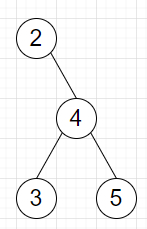# Program to find sum of all elements of a tree in Python

Suppose we have a binary tree containing some values, we have to find the sum of all values in the tree.

So, if the input is likethen the output will be 14

To solve this, we will follow these steps −

• Define a function recurse() . This will take node

• val := value of node

• if left of node is not null, then

• val := val + recurse(left of node)

• if right of node is not−null, then

• val := val + recurse(right of node)

• return val

• From the main method, do the following −

• if not root is non−zero, then

• return 0

• return recurse(root)

Let us see the following implementation to get better understanding −

## Example

Live Demo

class TreeNode:
def __init__(self, data, left = None, right = None):
self.val = data
self.left = left
self.right = right
class Solution:
def recurse(self, node):
val = node.val
if node.left:
val += self.recurse(node.left)
if node.right:
val += self.recurse(node.right)
return val
def solve(self, root):
if not root:
return 0
return self.recurse(root)
ob = Solution()
root = TreeNode(2)
root.right = TreeNode(4)
root.right.left = TreeNode(3)
root.right.right = TreeNode(5)
print(ob.solve(root))

## Input

root = TreeNode(2)
root.right = TreeNode(4)
root.right.left = TreeNode(3)
root.right.right = TreeNode(5)

## Output

14